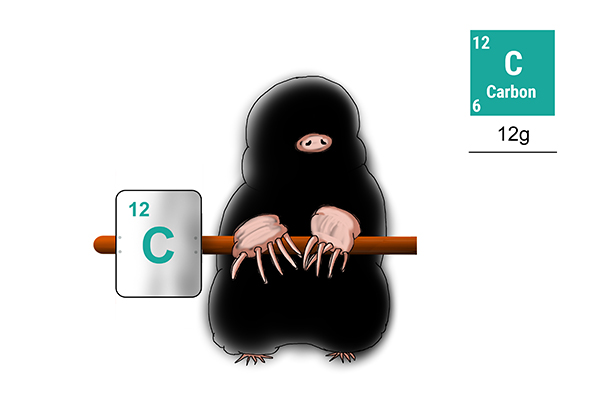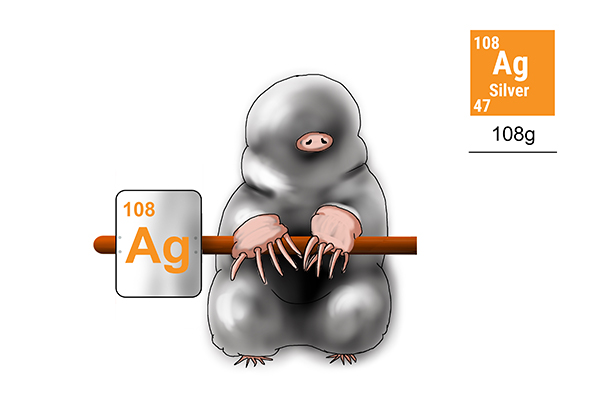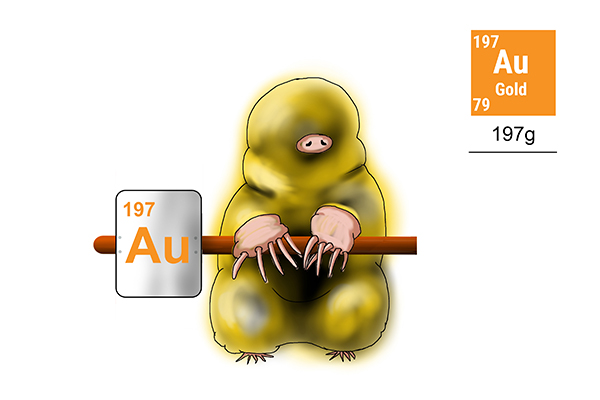# Moles: worked examples

### Magnesium

What is the mass of one mole of magnesium?

The real question is: what is the weight of 6 x 1023 atoms of magnesium?

To answer it, you’ll need to find the weight of one atom of magnesium and multiply by 6 x 1023.

What does one atom of magnesium weigh? How many protons and neutrons does it have? To work this out, look up the mass number of magnesium (24).

As there are 24 protons and neutrons in a magnesium atom, it will weigh: 24 x 1
6 x 1023
g

One mole of magnesium would weigh 6 x 1023 x 24 x 1
6 x 1023
g

6 x 1023 x 24 x 1
6 x 1023
= 24g

Answer: the mass of one mole of magnesium atoms is 24g.

Now we’ll find the mass of one mole of Carbon C, Silver Ag and Gold Au.

Remember, the real question is: what is the weight of 6 x 1023 atoms of carbon, silver or gold?

So find the weight of one atom of carbon, silver or gold and multiply by 6 x 1023.

### Carbon

To find the weight of 1 mole of carbon atoms, you first need to work out how many protons and neutrons it has (12).

1 proton or neutron weighs 1
6 x 1023
g

12 protons and neutrons = 12 x 1
6 x 1023
g

One mole of these = 6 x 1023 x 12 x  1
6 x 1023
= 12gAnswer: the weight of 1 mole of carbon = 12g

### Silver

To find the weight of 1 mole of silver atoms, first work out how many protons and neutrons silver has (108).

1 proton or neutron weighs 1
6 x 1023
g

108 protons and neutrons = 108 x 1
6 x 1023
g

One mole of these = 6 x 1023 x 108 x 1
6 x 1023
g = 108 gAnswer: the weight of 1 mole of silver is 108g

### Gold

To find the weight of 1 mole of gold atoms, find out how many protons and neutrons a gold atom has (197).

1 proton or neutron weighs1
6 x 1023
g

197 protons and neutrons = 197 x 1
6 x 1023
g

One mole of these = 6 x 1023 x 197 x 1
6 x 1023
g = 197gAnswer: the weight of 1 mole of gold is 197g

### Methane

What is the mass (weight) of 1 mole of CH4 (methane) molecules?

The real question is: what is the weight of 6 x 1023 atoms of one carbon atom and 4 hydrogen atoms?

How many protons and neutrons does carbon have: 12

How many protons and neutrons does 4 hydrogen atoms have: 4

12 + 4 = 16 protons and neutrons

1 proton or neutron weighs 1
6 x 1023
g

16 protons and neutrons weigh 16 x 1
6 x 1023
g

One mole = 6 x 1023 x 16 x 1
6 x 1023
g = 16g

Easy!  Aren’t moles easy!